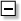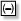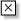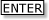Tapping into mathematics

Start this free course now. Just create an account and sign in. Enrol and complete the course for a free statement of participation or digital badge if available.

Free course

# Points to note

Here are a few points from the Exercise 1:

• The negative or minus sign for the answer 2 maybe slightly smaller and higher than the one used for subtraction in 5 − 7. There maybe two minus keys on your calculator keypad, as there are on the TI-84. The one which means do the operation subtract isand the one which shows a negative number is. So if you want to multiply 5 by 3, press: 53.

• The division sign may appear on the keyboard or on the display as /. Multiplication may be displayed as *.

• Decimal numbers less than 1 may be displayed without a 0 to the left of the decimal point.

• When you complete a calculation and then continue with an operation, your calculator will probably operate on the previous answer. In the case of the TI-84, it will indicate this by Ans. This is shown in answers (d) and (e).

• The true value of the fraction 5/7 goes on forever, but the calculator rounds off the answer and displays only ten or so digits. The TI-84 displays only 10 digits but it will store more (as is demonstrated when multiplying the answer of 5/7 by 7 and getting the answer 5).

• Division by 7 followed by multiplication by 7 results in the number being unchanged: 5 ÷ 7 × 7 is 5. This demonstrates the mathematical relationship between multiplication and division, namely that one is the inverse of the other. In other words, what one does, the other undoes. Two other operations which are inverses of each other are addition and subtraction.

• If you had divided the fraction 5/7 out by long division, you should get 0.71428571428571…. However because the calculator displays only a certain number of digits (ten in the case of the TI-84), it must make a sensible decision about how the final digit is displayed. The first ten digits would be 0.7142857142, but the TI-84 will display a 3 as the tenth digit, because the next digit is an 8, which means that the answer is nearer to 0.7142857143 than 0.7142857142.

The number of digits given in an answer by your calculator can usually be changed. For instance if you are dealing with money, you may only want 2 decimal places, as this is the norm in most currencies, e.g. £3.67, \$16.50.

MU120_4MCAL